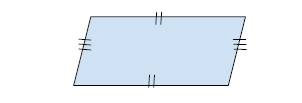# Program to find the Area of a Parallelogram in C++

In this problem, we are given two values that denote the base and height of a parallelogram. Our task is to create a Program to find the Area of a Parallelogram in C++.

Parallelogram is a four side closed figure that has opposite sides equal and parallel to each other.Let’s take an example to understand the problem,

## Input

B = 20, H = 15

## Output

300

## Explanation

Area of parallelogram = B * H = 20 * 15 = 300

## Solution Approach

To solve the problem, we will use the geometrical formula for area of parallelogram,

Area = base * height.

Program to illustrate the working of our solution,

## Example

Live Demo

#include <iostream>
using namespace std;
float calcParallelogramArea(float B, float H){
return (B * H);
}
int main() {
float B = 20, H = 15;
cout<<"The area of parallelogram with base "<<B<<" and height "<<H<<" is
"<<calcParallelogramArea(B, H);
return 0;
}

## Output

The area of parallelogram with base 20 and height 15 is 300